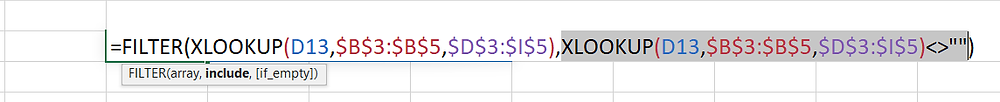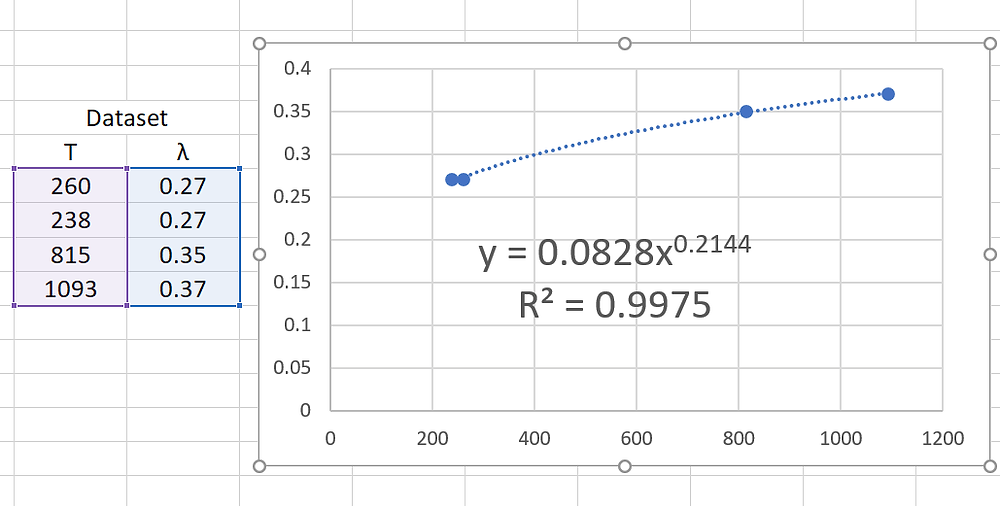# Modeling Thermal Conductivity of Insulation in Excel with Arrays

HOW USING ARRAYS CAN JUSTIFY A RAISE

This is a tutorial for some of the newer Excel formulas that manipulate arrays, with heat transfer calculations as the chosen example instead of banana inventory and employee salaries. A large part of performing heat transfer calculations is simply looking up intrinsic properties of the materials you are working with, and these functions make that easy. I hope to inspire someone to make efficient use of these features.

Example Problem: make an excel spreadsheet that calculates skin temperature with multiple types and layers of insulation.

Inputs: Hotface temperature, ambient conditions, wind speed, insulation type, and thicknesses

Output: Skin Temperature

Start by writing the steps to solve the problem before tackling the spreadsheet design, then follow these steps.

1) Curve Fit the thermal conductivity data of our insulation using a power function

2) Guess an initial temperature profile across the insulation

3) Calculate resistance of insulation layers at this temperature

4) Calculate a flux rate for this profile

5) Use flux rate as the basis for new initial temperatures

6) Repeat (which steps?) until Flux and Temperature do not change

We are only doing Step 1 for now. I will do Step 2 through 5 in another post if we get feedback that this one was useful. Remember, there are MANY ways to solve these problems in excel; this is just an example. This example adds a bit of extra complication to showcase more functions.

Here we have the table of data for our calculation.Let’s say we are interested in insulation A. To pull the temperature line of that data we use our first semi-new array function: XLOOKUP. This is where Excel masters may remember how HLOOKUP and VLOOKUP were limited for any non-simple case, but I assure you that the new XLOOKUP is lightyears ahead of its predecessors.With XLOOKUP you can return an entire row or column from a table as an array according to a lookup. In the above example, I look up the position of “A” in the first section and return the corresponding values in the second. You can do the same thing with INDEX with a nested MATCH function by not specifying a specific column in the INDEX:Notice that because of the blank spaces it has returned zeroes. These zeroes can cause problems, especially when we want to use the built in curve-fit functions. There are many remedies to this, but I am going to show how to use the Filter function.With the filter function, you can impose a limitation on the array. The first part of the formula selects the array and the second part says, ‘Array must not equal “” (blank)’.

Now you will see the blanks are filtered out and those two zeroes at the end are no longer part of the array:OK great, so we went through all that trouble and can automatically lookup properties. Now what? Now the Linest() function can be used without worry. If we tried to fit a power function with the extra zeroes from before it would have caused nothing but errors. In the example below we lookup both temperature and corresponding thermal conductivity using very similar formulas.The function Linest() is self-explanatory, but I will point out a few key tidbits about the cell references. The first is that I make use of a new selection symbol in excel to select the array: the pound sign. (I’m a nineties baby and grew up calling it pound, you may know # as the hash brown).

Why is this new reference type so useful? If you change the target insulation to B from A, there are more data points (6 of them), but the new array reference (‘#’) will automatically expand to catch those data points.

See Below:Automatic Adjustment Can be A Ray of Sunshine in Difficult Times

The next key point is that, since we want a power function curve fit, we must use the LOG10 of all the data points. You can do this by bracketing the array cell reference with LOG10(). This will result in b and log(a) terms for the function λ(T)=aT^b. We don’t want log(a); we want the ‘a’ term: a=10^log(a). And voila, you have a tool to look up and curve fit thermal conductivity. Just be sure not to overdo it on the sigfigs like I do.Set up a place to input Temperature and Return Lambda

Graph Verification:Proof of Accuracy: see it works!

Another useful function: sometimes when building spreadsheets, you will want arrays to be vertical instead of horizontal, and vice versa. This is where the transpose function comes in. It is quite self-explanatory:Transpose() changes the output from Horizontal to Vertical.

Recap:

The New array functions are great.

XLOOKUP() makes it easier to pull data.

FILTER() can be used to put criteria rules (like exclude blanks) in an array.

The ‘#’ can be used for array references.

TRANSPOSE() can shift arrays to how you need them.

I often end up using SEQUENCE() and INDEX() as well. I recommend you investigate those for additional functionality. I hope you learned something today. Thanks for reading!

Have an array of questions? Reach me at aj.sheikh@xrgtechnologies.com.

View the below spreadsheet for these calculations.

Sep 2020 Blog Example
.xlsx